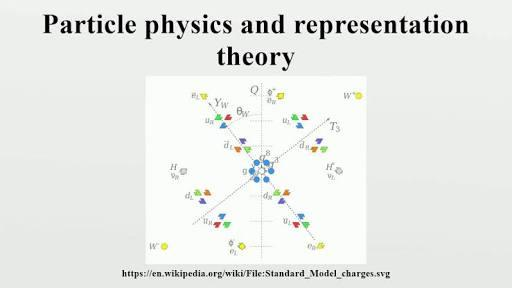# What Do You Know About Real Representations?

10 Questions | Total Attempts: 103SettingsIn mathematics--especially when considering the field of representation theory on a vector space represented by an invariant real structure, real representations are very useful. If you are willing to learn more about this theorem or get more information associated with it, consider this quiz and ensure that you practice the questions.

• 1.
How do we represent real vector space?
• A.

V

• B.

U

• C.

S

• D.

R

• 2.
How do we represent complex vector space?
• A.

V

• B.

U

• C.

S

• D.

R

• 3.
What is the invariant real structure also termed as?
• A.

Antilinear equivariant map

• B.

Linear equivariant map

• C.

Stability

• D.

Control of vehicles flying through air

• 4.
In the subject of physics, representations are viewed as what?
• A.

Matrices

• B.

Momentum

• C.

Inertia

• D.

Space

• 5.
What happens to the direct sum of quaternionic and real representation?
• A.

Real

• B.

Neither real nor quaternionic

• C.

Both real and quaternionic

• D.

Quaternionic

• 6.
A representation on a complex vector space is isomorphic to what?
• A.

Dual representation

• B.

Single representation

• C.

Complete representation

• D.

Multiple representation

• 7.
What is the descriptive feature of a Hermitian form?
• A.

Representation changes to a degenerate invariant linear form

• B.

Representation changes to a nondegenerate invariant sesquilinear form

• C.

Representation changes to the linear form

• D.

Representation changes to the sesquilinear form

• 8.
The representation of a Hermitian form is also referred to as what?
• A.

Pseudo hermitian

• B.

Real hermitian

• C.

Universal hermitian

• D.

Concurrent hermitian

• 9.
The representation of symmetric groups are...
• A.

Irrational

• B.

Real

• C.

Complex

• D.

Rational

• 10.
The representation of rotational groups are...
• A.

Complex

• B.

Rational

• C.

Irrational

• D.

Real

Related TopicsBack to top